Publicité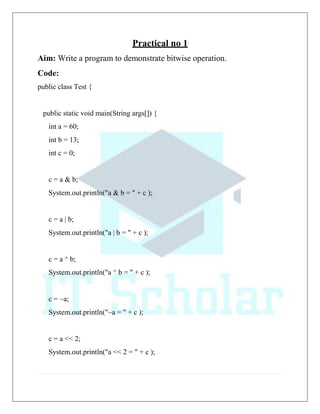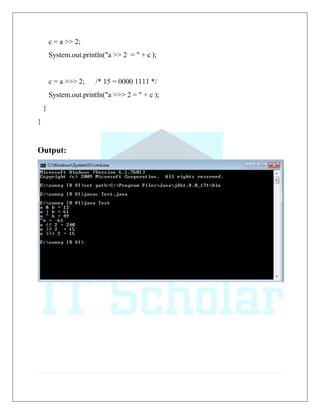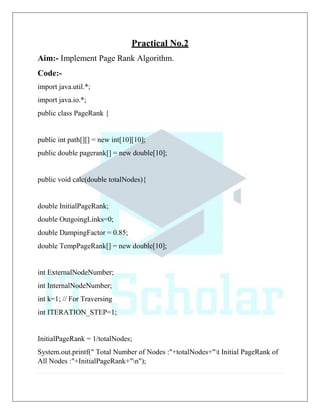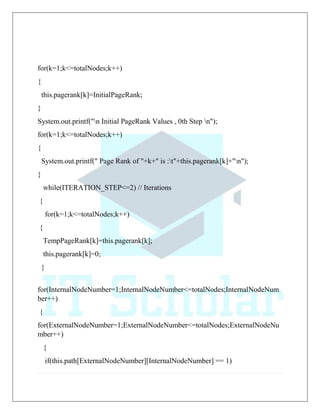Publicité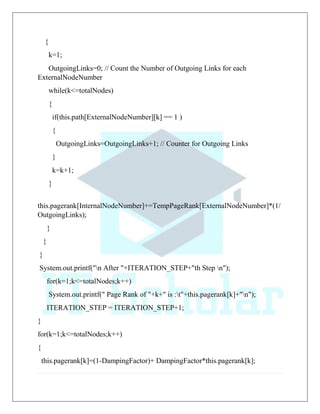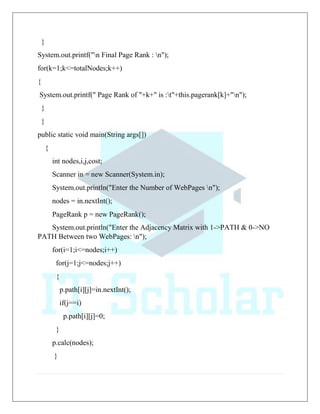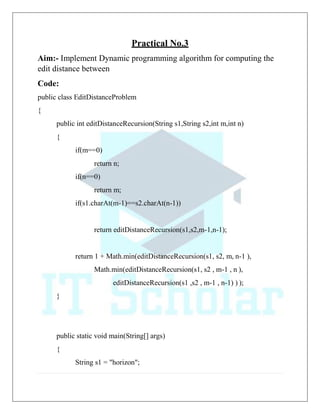Publicité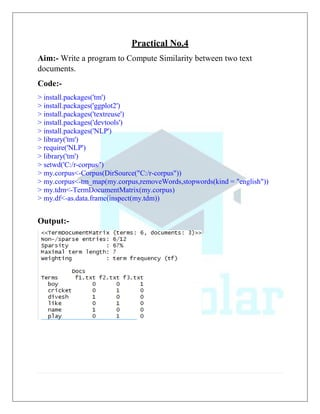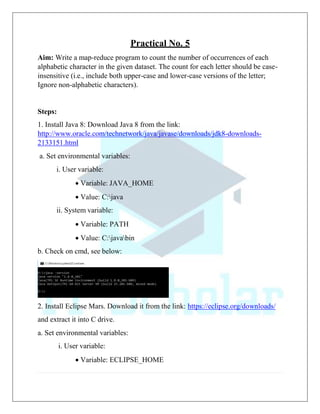Publicité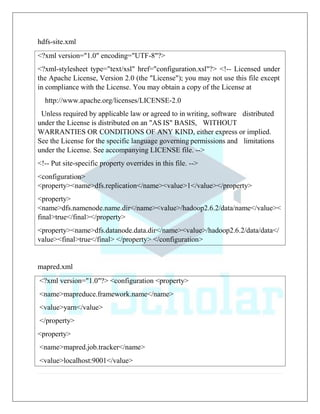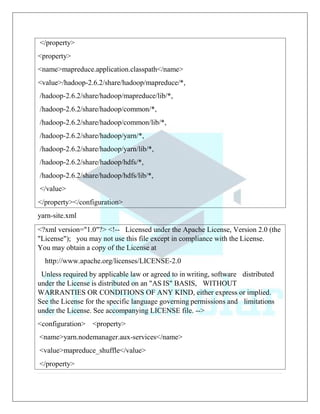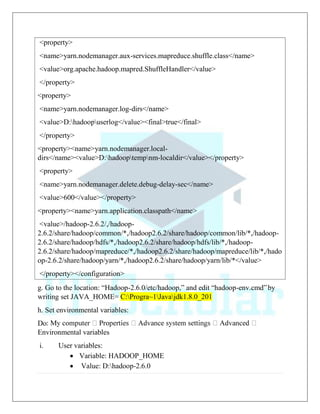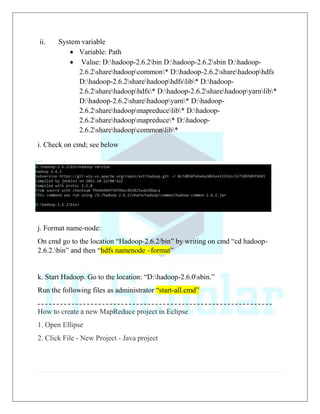Publicité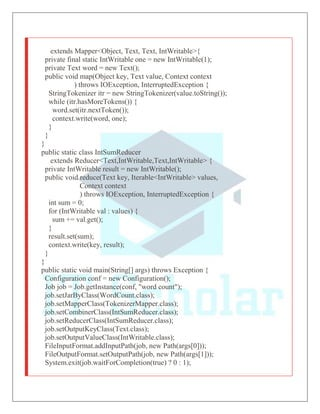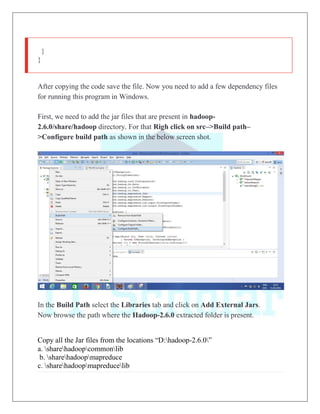Publicité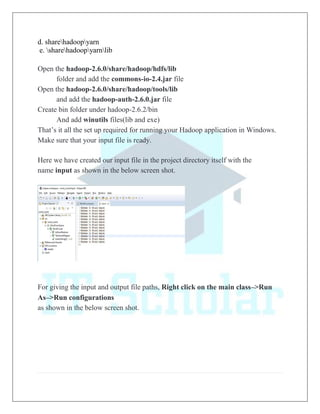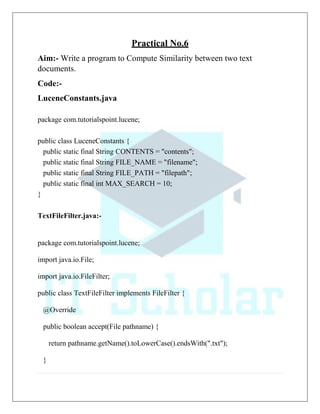Publicité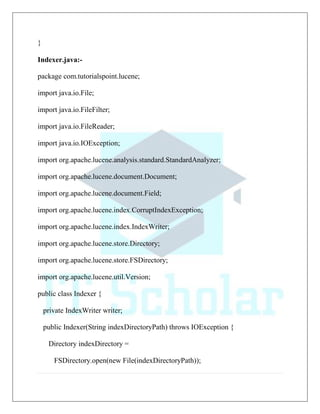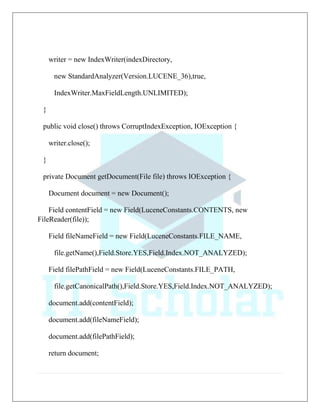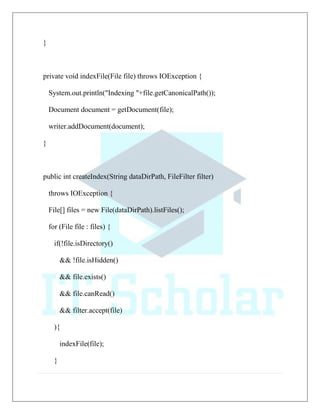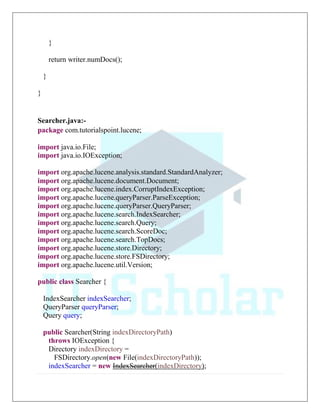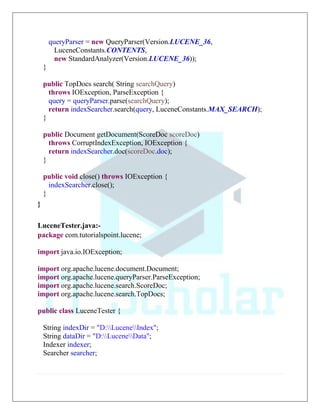Publicité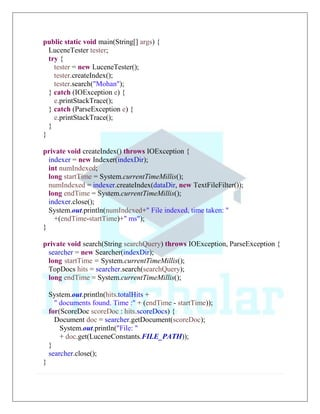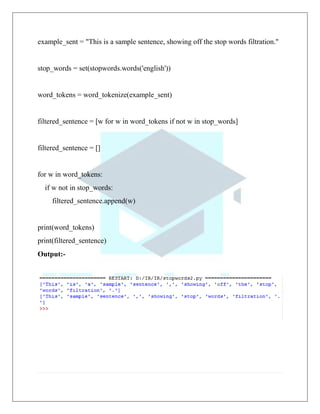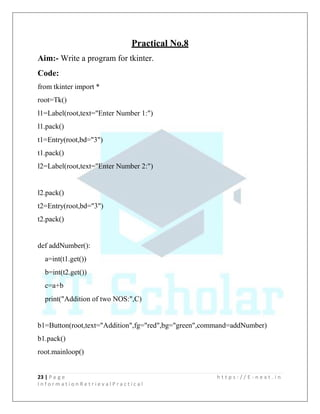Publicité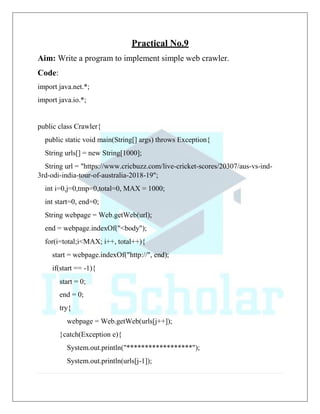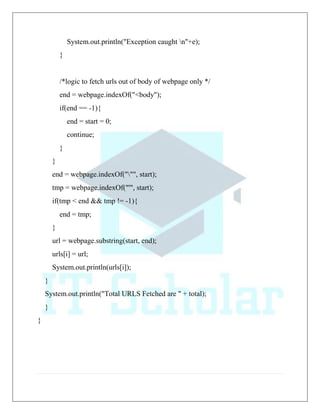Publicité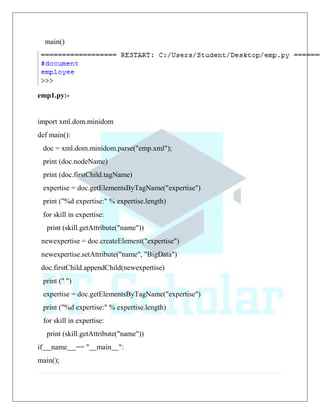Prochain SlideShareBurn down the silos! Helping dev and ops gel on high availability websites
Chargement dans ... 3
1 sur 47
Publicité

### IR Journal (itscholar.codegency.co.in).pdf

1. Practical no 1 Aim: Write a program to demonstrate bitwise operation. Code: public class Test { public static void main(String args[]) { int a = 60; int b = 13; int c = 0; c = a & b; System.out.println("a & b = " + c ); c = a | b; System.out.println("a | b = " + c ); c = a ^ b; System.out.println("a ^ b = " + c ); c = ~a; System.out.println("~a = " + c ); c = a << 2; System.out.println("a << 2 = " + c );
2. c = a >> 2; System.out.println("a >> 2 = " + c ); c = a >>> 2; /* 15 = 0000 1111 */ System.out.println("a >>> 2 = " + c ); } } Output:
3. Practical No.2 Aim:- Implement Page Rank Algorithm. Code:- import java.util.*; import java.io.*; public class PageRank { public int path[][] = new int; public double pagerank[] = new double; public void calc(double totalNodes){ double InitialPageRank; double OutgoingLinks=0; double DampingFactor = 0.85; double TempPageRank[] = new double; int ExternalNodeNumber; int InternalNodeNumber; int k=1; // For Traversing int ITERATION_STEP=1; InitialPageRank = 1/totalNodes; System.out.printf(" Total Number of Nodes :"+totalNodes+"t Initial PageRank of All Nodes :"+InitialPageRank+"n");
4. for(k=1;k<=totalNodes;k++) { this.pagerank[k]=InitialPageRank; } System.out.printf("n Initial PageRank Values , 0th Step n"); for(k=1;k<=totalNodes;k++) { System.out.printf(" Page Rank of "+k+" is :t"+this.pagerank[k]+"n"); } while(ITERATION_STEP<=2) // Iterations { for(k=1;k<=totalNodes;k++) { TempPageRank[k]=this.pagerank[k]; this.pagerank[k]=0; } for(InternalNodeNumber=1;InternalNodeNumber<=totalNodes;InternalNodeNum ber++) { for(ExternalNodeNumber=1;ExternalNodeNumber<=totalNodes;ExternalNodeNu mber++) { if(this.path[ExternalNodeNumber][InternalNodeNumber] == 1)
5. { k=1; OutgoingLinks=0; // Count the Number of Outgoing Links for each ExternalNodeNumber while(k<=totalNodes) { if(this.path[ExternalNodeNumber][k] == 1 ) { OutgoingLinks=OutgoingLinks+1; // Counter for Outgoing Links } k=k+1; } this.pagerank[InternalNodeNumber]+=TempPageRank[ExternalNodeNumber]*(1/ OutgoingLinks); } } } System.out.printf("n After "+ITERATION_STEP+"th Step n"); for(k=1;k<=totalNodes;k++) System.out.printf(" Page Rank of "+k+" is :t"+this.pagerank[k]+"n"); ITERATION_STEP = ITERATION_STEP+1; } for(k=1;k<=totalNodes;k++) { this.pagerank[k]=(1-DampingFactor)+ DampingFactor*this.pagerank[k];
6. } System.out.printf("n Final Page Rank : n"); for(k=1;k<=totalNodes;k++) { System.out.printf(" Page Rank of "+k+" is :t"+this.pagerank[k]+"n"); } } public static void main(String args[]) { int nodes,i,j,cost; Scanner in = new Scanner(System.in); System.out.println("Enter the Number of WebPages n"); nodes = in.nextInt(); PageRank p = new PageRank(); System.out.println("Enter the Adjacency Matrix with 1->PATH & 0->NO PATH Between two WebPages: n"); for(i=1;i<=nodes;i++) for(j=1;j<=nodes;j++) { p.path[i][j]=in.nextInt(); if(j==i) p.path[i][j]=0; } p.calc(nodes); }
7. } Output:-
8. Practical No.3 Aim:- Implement Dynamic programming algorithm for computing the edit distance between Code: public class EditDistanceProblem { public int editDistanceRecursion(String s1,String s2,int m,int n) { if(m==0) return n; if(n==0) return m; if(s1.charAt(m-1)==s2.charAt(n-1)) return editDistanceRecursion(s1,s2,m-1,n-1); return 1 + Math.min(editDistanceRecursion(s1, s2, m, n-1 ), Math.min(editDistanceRecursion(s1, s2 , m-1 , n ), editDistanceRecursion(s1 ,s2 , m-1 , n-1) ) ); } public static void main(String[] args) { String s1 = "horizon";
9. String s2 = "horizontal"; EditDistanceProblem ed = new EditDistanceProblem(); System.out.println("Minimum Edit Distance - (Recursion): " + ed.editDistanceRecursion(s1,s2,s1.length(),s2.length() ) ); } } Output:
10. Practical No.4 Aim:- Write a program to Compute Similarity between two text documents. Code:- > install.packages('tm') > install.packages('ggplot2') > install.packages('textreuse') > install.packages('devtools') > install.packages('NLP') > library('tm') > require('NLP') > library('tm') > setwd('C:/r-corpus/') > my.corpus<-Corpus(DirSource("C:/r-corpus")) > my.corpus<-tm_map(my.corpus,removeWords,stopwords(kind = "english")) > my.tdm<-TermDocumentMatrix(my.corpus) > my.df<-as.data.frame(inspect(my.tdm)) Output:-
11. > barplot(as.matrix(my.tdm))
19. Hereafter clicking on the New Java project, it will ask for the project name as shown in the below screen shot. Give a project name. Here we have given the project name as Word_count.
20. Now after giving the project name, a project will be created with the given name. Click on the project and inside the project, you will find a directory called src. Right click and create new class as shown in the below screen shot.
21. Now you will be prompted with another screen to provide the class name as shown in the below screen shot. Here, give the class name of your choice. We have given the name as WordCount. Inside the src, a file with name WordCount.java has been created. Click on the file and write the MapReduce code for the word count program
23. extends Mapper<Object, Text, Text, IntWritable>{ private final static IntWritable one = new IntWritable(1); private Text word = new Text(); public void map(Object key, Text value, Context context ) throws IOException, InterruptedException { StringTokenizer itr = new StringTokenizer(value.toString()); while (itr.hasMoreTokens()) { word.set(itr.nextToken()); context.write(word, one); } } } public static class IntSumReducer extends Reducer<Text,IntWritable,Text,IntWritable> { private IntWritable result = new IntWritable(); public void reduce(Text key, Iterable<IntWritable> values, Context context ) throws IOException, InterruptedException { int sum = 0; for (IntWritable val : values) { sum += val.get(); } result.set(sum); context.write(key, result); } } public static void main(String[] args) throws Exception { Configuration conf = new Configuration(); Job job = Job.getInstance(conf, "word count"); job.setJarByClass(WordCount.class); job.setMapperClass(TokenizerMapper.class); job.setCombinerClass(IntSumReducer.class); job.setReducerClass(IntSumReducer.class); job.setOutputKeyClass(Text.class); job.setOutputValueClass(IntWritable.class); FileInputFormat.addInputPath(job, new Path(args)); FileOutputFormat.setOutputPath(job, new Path(args)); System.exit(job.waitForCompletion(true) ? 0 : 1);
24. After copying the code save the file. Now you need to add a few dependency files for running this program in Windows. First, we need to add the jar files that are present in hadoop- 2.6.0/share/hadoop directory. For that Righ click on src–>Build path– >Configure build path as shown in the below screen shot. In the Build Path select the Libraries tab and click on Add External Jars. Now browse the path where the Hadoop-2.6.0 extracted folder is present. Copy all the Jar files from the locations “D:hadoop-2.6.0” a. sharehadoopcommonlib b. sharehadoopmapreduce c. sharehadoopmapreducelib } }
26. In the main select the project name and the class name of the program as shown in the below screen shot.
27. Now move into the Arguments tab and provide the input file path and the output file path as shown in the below screen shot. Since we have our input file inside the project directory itself, we have just given inp as input file path and then a tabspace. We have given the output file path as just output. It will create the output directory inside the project directory itself.
28. Now click on Run. You will see the Eclipse console running
29. Practical No.6 Aim:- Write a program to Compute Similarity between two text documents. Code:- LuceneConstants.java package com.tutorialspoint.lucene; public class LuceneConstants { public static final String CONTENTS = "contents"; public static final String FILE_NAME = "filename"; public static final String FILE_PATH = "filepath"; public static final int MAX_SEARCH = 10; } TextFileFilter.java:- package com.tutorialspoint.lucene; import java.io.File; import java.io.FileFilter; public class TextFileFilter implements FileFilter { @Override public boolean accept(File pathname) { return pathname.getName().toLowerCase().endsWith(".txt"); }
30. } Indexer.java:- package com.tutorialspoint.lucene; import java.io.File; import java.io.FileFilter; import java.io.FileReader; import java.io.IOException; import org.apache.lucene.analysis.standard.StandardAnalyzer; import org.apache.lucene.document.Document; import org.apache.lucene.document.Field; import org.apache.lucene.index.CorruptIndexException; import org.apache.lucene.index.IndexWriter; import org.apache.lucene.store.Directory; import org.apache.lucene.store.FSDirectory; import org.apache.lucene.util.Version; public class Indexer { private IndexWriter writer; public Indexer(String indexDirectoryPath) throws IOException { Directory indexDirectory = FSDirectory.open(new File(indexDirectoryPath));
31. writer = new IndexWriter(indexDirectory, new StandardAnalyzer(Version.LUCENE_36),true, IndexWriter.MaxFieldLength.UNLIMITED); } public void close() throws CorruptIndexException, IOException { writer.close(); } private Document getDocument(File file) throws IOException { Document document = new Document(); Field contentField = new Field(LuceneConstants.CONTENTS, new FileReader(file)); Field fileNameField = new Field(LuceneConstants.FILE_NAME, file.getName(),Field.Store.YES,Field.Index.NOT_ANALYZED); Field filePathField = new Field(LuceneConstants.FILE_PATH, file.getCanonicalPath(),Field.Store.YES,Field.Index.NOT_ANALYZED); document.add(contentField); document.add(fileNameField); document.add(filePathField); return document;
32. } private void indexFile(File file) throws IOException { System.out.println("Indexing "+file.getCanonicalPath()); Document document = getDocument(file); writer.addDocument(document); } public int createIndex(String dataDirPath, FileFilter filter) throws IOException { File[] files = new File(dataDirPath).listFiles(); for (File file : files) { if(!file.isDirectory() && !file.isHidden() && file.exists() && file.canRead() && filter.accept(file) ){ indexFile(file); }
33. } return writer.numDocs(); } } Searcher.java:- package com.tutorialspoint.lucene; import java.io.File; import java.io.IOException; import org.apache.lucene.analysis.standard.StandardAnalyzer; import org.apache.lucene.document.Document; import org.apache.lucene.index.CorruptIndexException; import org.apache.lucene.queryParser.ParseException; import org.apache.lucene.queryParser.QueryParser; import org.apache.lucene.search.IndexSearcher; import org.apache.lucene.search.Query; import org.apache.lucene.search.ScoreDoc; import org.apache.lucene.search.TopDocs; import org.apache.lucene.store.Directory; import org.apache.lucene.store.FSDirectory; import org.apache.lucene.util.Version; public class Searcher { IndexSearcher indexSearcher; QueryParser queryParser; Query query; public Searcher(String indexDirectoryPath) throws IOException { Directory indexDirectory = FSDirectory.open(new File(indexDirectoryPath)); indexSearcher = new IndexSearcher(indexDirectory);
34. queryParser = new QueryParser(Version.LUCENE_36, LuceneConstants.CONTENTS, new StandardAnalyzer(Version.LUCENE_36)); } public TopDocs search( String searchQuery) throws IOException, ParseException { query = queryParser.parse(searchQuery); return indexSearcher.search(query, LuceneConstants.MAX_SEARCH); } public Document getDocument(ScoreDoc scoreDoc) throws CorruptIndexException, IOException { return indexSearcher.doc(scoreDoc.doc); } public void close() throws IOException { indexSearcher.close(); } } LuceneTester.java:- package com.tutorialspoint.lucene; import java.io.IOException; import org.apache.lucene.document.Document; import org.apache.lucene.queryParser.ParseException; import org.apache.lucene.search.ScoreDoc; import org.apache.lucene.search.TopDocs; public class LuceneTester { String indexDir = "D:LuceneIndex"; String dataDir = "D:LuceneData"; Indexer indexer; Searcher searcher;
35. public static void main(String[] args) { LuceneTester tester; try { tester = new LuceneTester(); tester.createIndex(); tester.search("Mohan"); } catch (IOException e) { e.printStackTrace(); } catch (ParseException e) { e.printStackTrace(); } } private void createIndex() throws IOException { indexer = new Indexer(indexDir); int numIndexed; long startTime = System.currentTimeMillis(); numIndexed = indexer.createIndex(dataDir, new TextFileFilter()); long endTime = System.currentTimeMillis(); indexer.close(); System.out.println(numIndexed+" File indexed, time taken: " +(endTime-startTime)+" ms"); } private void search(String searchQuery) throws IOException, ParseException { searcher = new Searcher(indexDir); long startTime = System.currentTimeMillis(); TopDocs hits = searcher.search(searchQuery); long endTime = System.currentTimeMillis(); System.out.println(hits.totalHits + " documents found. Time :" + (endTime - startTime)); for(ScoreDoc scoreDoc : hits.scoreDocs) { Document doc = searcher.getDocument(scoreDoc); System.out.println("File: " + doc.get(LuceneConstants.FILE_PATH)); } searcher.close(); }
36. } Output:-
37. Practical No.7 Aim:- Write a program for Pre-processing of a Text Document: stop word removal. Stopwords1.py:- >>> import nltk >>> from nltk.corpus import stopwords >>> set(stopwords.words('english')) Output:- Stopwords1.py:- from nltk.corpus import stopwords from nltk.tokenize import word_tokenize
38. example_sent = "This is a sample sentence, showing off the stop words filtration." stop_words = set(stopwords.words('english')) word_tokens = word_tokenize(example_sent) filtered_sentence = [w for w in word_tokens if not w in stop_words] filtered_sentence = [] for w in word_tokens: if w not in stop_words: filtered_sentence.append(w) print(word_tokens) print(filtered_sentence) Output:-
39. Practical No.8 Aim:- Write a program for tkinter. Code: from tkinter import * root=Tk() l1=Label(root,text="Enter Number 1:") l1.pack() t1=Entry(root,bd="3") t1.pack() l2=Label(root,text="Enter Number 2:") l2.pack() t2=Entry(root,bd="3") t2.pack() def addNumber(): a=int(t1.get()) b=int(t2.get()) c=a+b print("Addition of two NOS:",C) b1=Button(root,text="Addition",fg="red",bg="green",command=addNumber) b1.pack() root.mainloop() 23 | P a g e h t t p s : / / E - n e x t . i n I n f o r m a t i o n R e t r i e v a l P r a c t i c a l
40. Output:-
41. Practical No.9 Aim: Write a program to implement simple web crawler. Code: import java.net.*; import java.io.*; public class Crawler{ public static void main(String[] args) throws Exception{ String urls[] = new String; String url = "https://www.cricbuzz.com/live-cricket-scores/20307/aus-vs-ind- 3rd-odi-india-tour-of-australia-2018-19"; int i=0,j=0,tmp=0,total=0, MAX = 1000; int start=0, end=0; String webpage = Web.getWeb(url); end = webpage.indexOf("<body"); for(i=total;i<MAX; i++, total++){ start = webpage.indexOf("http://", end); if(start == -1){ start = 0; end = 0; try{ webpage = Web.getWeb(urls[j++]); }catch(Exception e){ System.out.println("******************"); System.out.println(urls[j-1]);
42. System.out.println("Exception caught n"+e); } /*logic to fetch urls out of body of webpage only */ end = webpage.indexOf("<body"); if(end == -1){ end = start = 0; continue; } } end = webpage.indexOf(""", start); tmp = webpage.indexOf("'", start); if(tmp < end && tmp != -1){ end = tmp; } url = webpage.substring(start, end); urls[i] = url; System.out.println(urls[i]); } System.out.println("Total URLS Fetched are " + total); } }
43. /*This class contains a static function which will fetch the webpage of the given url and return as a string */ class Web{ public static String getWeb(String address)throws Exception{ String webpage = ""; String inputLine = ""; URL url = new URL(address); BufferedReader in = new BufferedReader( new InputStreamReader(url.openStream())); while ((inputLine = in.readLine()) != null) webpage += inputLine; in.close(); return webpage; } } Output:
44. Practical No.10 Aim:- Write a program to parse XML text, generate Web graph and compute topic specific page rank. emp.xml:- <?xml version="1.0" encoding="UTF-8"?> <employee> <fname>Divesh</fname> <lname>Saurabh</lname> <home>Thane</home> <expertise name="SQl"/> <expertise name="Python"/> <expertise name="Testing"/> <expertise name="Business"/> </employee> emp.py:- import xml.dom.minidom def main(): doc=xml.dom.minidom.parse("emp.xml"); print(doc.nodeName) print(doc.firstChild.tagName) if name ==" main ":
45. main() emp1.py:- import xml.dom.minidom def main(): doc = xml.dom.minidom.parse("emp.xml"); print (doc.nodeName) print (doc.firstChild.tagName) expertise = doc.getElementsByTagName("expertise") print ("%d expertise:" % expertise.length) for skill in expertise: print (skill.getAttribute("name")) newexpertise = doc.createElement("expertise") newexpertise.setAttribute("name", "BigData") doc.firstChild.appendChild(newexpertise) print (" ") expertise = doc.getElementsByTagName("expertise") print ("%d expertise:" % expertise.length) for skill in expertise: print (skill.getAttribute("name")) if name == " main ": main();
46. Output:
Publicité